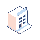• Pricing

# Relation filed

Hello

I want to create a relation field from products to currency rate

company currency is AED and want to see USD rate in the new filed i did like this:

relation = currency_id.inverse_rate but the rate is 1 and its comany rate how can i kink it USD rate?To create a relation field from products to currency rate, you can use the currency_id field on the product.product model, which represents the currency of the product. You can then define a related field that gets the USD rate for that currency.

Here's an example of how you can define the related field:

from odoo import fields, models

class ProductProduct(models.Model):
_inherit = 'product.product'

usd_rate = fields.Float(string='USD Rate', compute='_compute_usd_rate', store=True)

def _compute_usd_rate(self):
for product in self:
currency = product.currency_id
if currency:
usd_currency = self.env['res.currency'].search([('name', '=', 'USD')], limit=1)
if usd_currency:
usd_rate = currency._convert(1, usd_currency, product.company_id, fields.Date.today())
product.usd_rate = usd_rate

ThanksDear Mahijaban
company currency in fact is USD and i need to computed based on AED and here is my code but its not working. i put this code in compute section of the field

class ProductProduct(models.Model):
_inherit = 'product.product'

x_studio_today_rate_aed = fields.Float(string='Today Rate (AED)', compute='_compute_x_studio_today_rate_aed', store=True)

def _compute_x_studio_today_rate_aed(self):
for product in self:
currency = product.currency_id
if currency:
x_studio_today_rate_aed = self.env['res.currency'].search([('name', '=', 'AED')], limit=1)
if x_studio_today_rate_aed:
x_studio_today_rate_aed = currency._convert(1, x_studio_today_rate_aed, product.company_id, fields.Date.today())
product.x_studio_today_rate_aed = x_studio_today_rate_aed

Dependencies = x_studio_today_rate_aed

i was wondering if might be able to correct me

class ProductProduct(models.Model):
_inherit = 'product.product'

x_studio_today_rate_aed = fields.Float(string='Today Rate (AED)', compute='_compute_x_studio_today_rate_aed', store=True)

@api.depends('currency_id', 'company_id.currency_id', 'company_id.currency_id.rate_ids')
def _compute_x_studio_today_rate_aed(self):
for product in self:
currency = product.currency_id
if currency:
aed_currency = self.env['res.currency'].search([('name', '=', 'AED')], limit=1)
if aed_currency:
today_rate = aed_currency._get_conversion_rate(currency, product.company_id.currency_id, fields.Date.today())
product.x_studio_today_rate_aed = today_rate